American Journal of Modern Physics
Volume 6, Issue 1, January 2017, Pages: 1-9

Optical and Electrical Modeling of Dye Sensitized Solar Cell: Influence of the Overlap Distance Between TiO2 Particles

El Hadji Oumar Gueye*, Papa Douta Tall, Kharouna Talla, Abdoulaye Ndiaye Dione, Allé Dioum, Mouhamadou Bachir Gaye, Ndeye Maty Ndiaye, Balla Diop Ngom, Aboubaker Chedikh Beye

Laboratory of Solid State Physics and Materials Science, Faculty of Sciences et Techniques, Cheikh Anta Diop University, Dakar, Senegal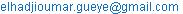(E. H. O. Gueye)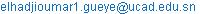(E. H. O. Gueye)

*Corresponding author

El Hadji Oumar Gueye, Papa Douta Tall, Kharouna Talla, Abdoulaye Ndiaye Dione, Allé Dioum, Mouhamadou Bachir Gaye, Ndeye Maty Ndiaye, Balla Diop Ngom, Aboubaker Chedikh Beye. Optical and Electrical Modeling of Dye Sensitized Solar Cell: Influence of the Overlap Distance Between TiO2 Particles. American Journal of Modern Physics. Vol. 6, No. 1, 2017, pp. 1-9. doi: 10.11648/j.ajmp.20170601.11

Received: January 3, 2017; Accepted: January 12, 2017; Published: February 3, 2017

Abstract: Dye sensitized solar cells (DSSC) are used for photovoltaic applications. The paper presents a methodology for optical and electrical modeling of dye-sensitized solar cells (DSSCs). In order to take into account the influence of the overlap distance between two TiO2 particles on the cell an optoelectronic model for DSSC is presented in this paper. From the radiative transfer equation and Mie theory, the optical generation rate of cell is deduced. Coupling the output of the optical model (the dye absorption rate) to an electrical model allows determination of short-circuit current density and maximum power output. Due to our model, the dependence effects of the overlap distance upon the porosity, the optical generation rate, the short circuit current density and the maximum power output are evidenced. Thus, we see that when the overlap distance increases the porosity decreases. In addition, when the overlap distance increases, the absorption rate decreases when the overlap distance is greater than TiO2 radius divide by 5. Moreover, we see that when the overlap distance is lower than the TiO2 radius divided by 5, the short circuit current density and the maximum power output increase. However, when the overlap distance is higher than TiO2 radius divided by 5 they decrease. Thus, according to the model, we see that the optimal overlap distance is equal to TiO2 radius divide by 5. Our results agree with those found in the literature.

Keywords: Dye-Sensitized Solar Cell, Optical Parameters, Electrical Parameters, Overlap Distance, TiO2 Radius

Contents

1. Introduction

Dye sensitized solar cells (DSCs) as alternative to solar cells have been widely studied in recent years [1-3]. Record efficiencies of over 11% have been achieved with ruthenium-complex sensitizers on laboratory-scale devices. [4-6] Dye sensitized solar cell is a mixture of nanostructured films, a sensitizer and an electrolyte containing the mediator sandwiched between two electrodes: a conductive oxide as an anode and a counter electrode as cathode as depicted in figure 1. The efficiency of absorption of the incoming light by the dye is one of the paramount parameters for the cell performance. Accordingly, the peculiar composition of the photoactive layer makes the dye-sensitized solar cells very complicated to establish an optoelectronic model. Indeed, it is composed of a mixture of three materials (titanium dioxide, dye molecules and tri-iodide ions immerged within the electrolyte) which could raise homogeneity problems.

Various optical [7-8], electrical [9-18] and optoelectronic model [19-20] have been developed.

The purpose of our study is to present an optoelectronic model that take into account the influence of overlap distance on dye sensitive solar cell.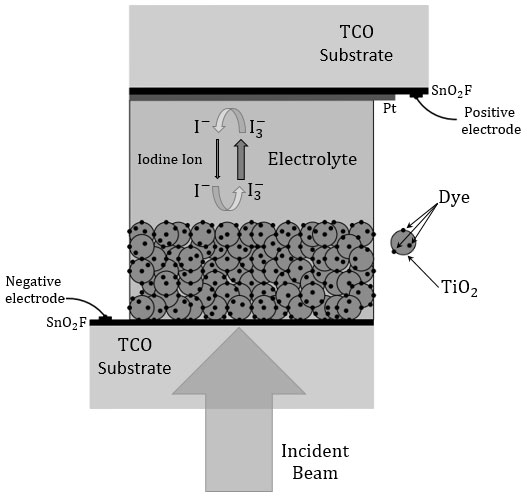Figure 1. Structure of the Dye sensitized solar cell.

2. Computational Model

2.1. Optical Model

The model considered is depicted in figure 2. Where x represents the depth of the photoactive layer. Thus, our study is essentially limited to the photoactive layer sandwiched between the two electrodes. The photoactive layer is a mixture of Titanium dioxide (TiO2), a dye (Z907), a mediator and an electrolyte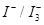.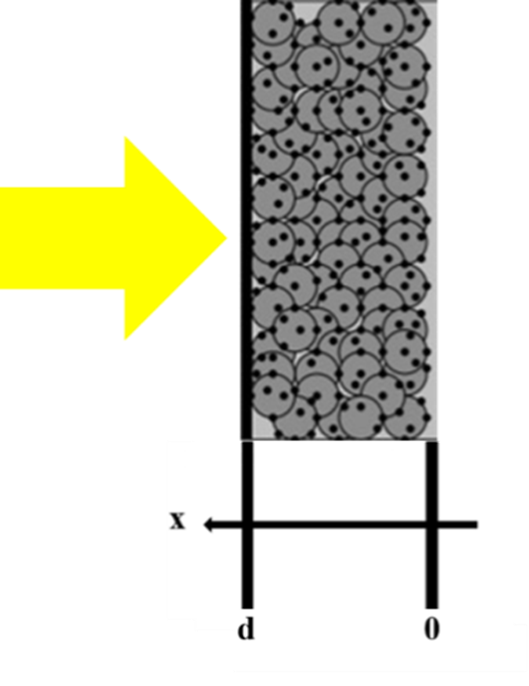Figure 2. Schematic of the photoactive layer.

First, we calculate the scattering cross sections and absorption through the Mie theory in the case of a mono-dispersed particle size. Our medium is composed of three compounds; we calculate the scattering cross sections for the nanostructured film (TiO2 here) and the electrolyte / mediator. Indeed, the particle size of dyes is low , they do not participate in the scattering process. Then macroscopic scattering and absorption coefficient s and k will be calculated for each compound.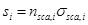(1)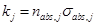(2)

Where i = electrolyte/mediator or TiO2; j = dye or electrolyte /mediator. The particle density of the electrolytic/ mediator will be given by the equation: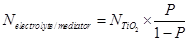(3)

With P the porosity.

The hypothesis we making here is to consider the TIO2/Dye as one particle. Since the diffusion of the dyes particles is very low we consider that the particle (TiO2/Dye) have the same dimensions as the simple TiO2 particle . On the other hands since the TiO2 particle doesn't absorb we consider also that the absorption of the particle (TiO2/Dye) is just coming from the simple dye particle Therefore we have: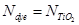(4)

Then: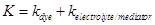(5)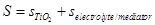(6)

To determine the porosity, we define the system as follows: First, the radiuses of all the nanoparticles are the same. Colloids are considered perfectly spherical. The penetration length for two neighboring particles is given by: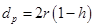(7)

Where dp: distance between nanoparticles, r: radius of the sphere, h: the overlap distance

Equation (7) can be visualized in figure 3.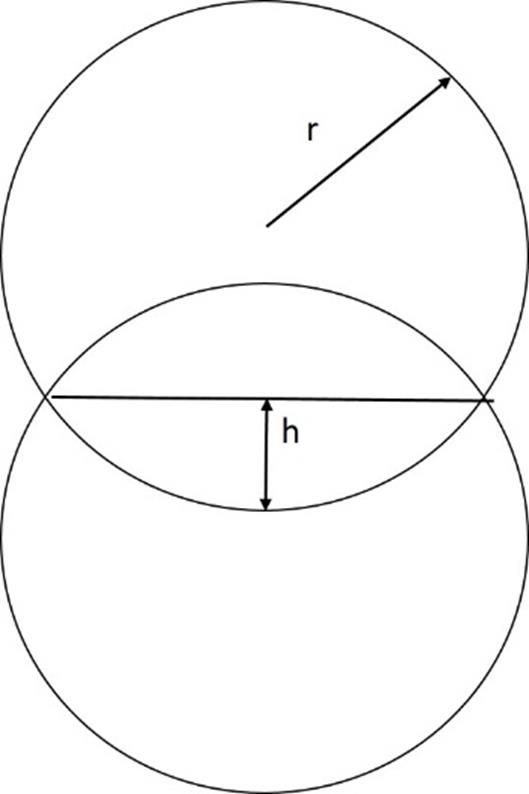Figure 3. Schematic picture of the overlap between two particles.

Taylor et al's equations are used to determine the porosity [21-22]. In these equations, the porosity is deduced by determining the volume of a single cell and the solid volume (sphere in our case) in a single cell.

The volume of the single cell is a function of the packing factor and diameter of the sphere. The volume is: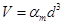(8)

αm: packing factor, d: diameter of the sphere.

With the volume of the single cell known, the calculation of the porosity requires the determination of the total volume of the sphere in the single cell. For this calculation, Taylor et al based themselves on the calculations of Deb . It determines the volume of a sphere taking into account the overlapping surface between the spheres. Thus, the volume of the sphere in the single cell is: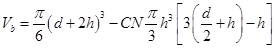(9)

h: overlap distance; CN: coordination number

The first term on the right corresponds to the total volume of the sphere. The second term corresponds to the sum of the volumes of each spherical cape. Taylor et al. [21-22] used equations for the volume of single cell (6), the volume of sphere (7), and then obtained the following equation for the calculation of the porosity of a cell containing a number n of spheres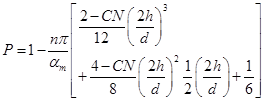(10)

We will calculate the porosity for a diamond-like structure using equation (8) and Table 1.

Table 1. Characteristic of diamond like structure.

 Structure Coordination number Number of spheres by unit cell Packing factor αm Diamond 4 8 12.35

Afterwards the macroscopic coefficients K and S are used to calculate the collimated flux (Ic and Jc) and diffused flux (Id and Jd). Basically, the 4-flux approximation of the radiative transfer equation  given by equations. (7, 8, 9, 10) was considered. These different fluxes are illustrated in figure 3. In fact, the variation of the flows in the medium with an infinitesimal value of the thickness dx can be established using the equilibrium between the scattering and the absorption energy in forward and backward.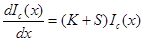(11)(12)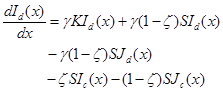(13)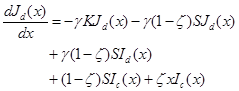(14)

The factor γ is the equivalent path length for the diffuse fluxes, which ranges from 1 for collimated light to 2 for isotropic scattered light . Thus, the analytical expression of the forward scattering ratio (ζ) is obtained from C. Rozé et al. analysis .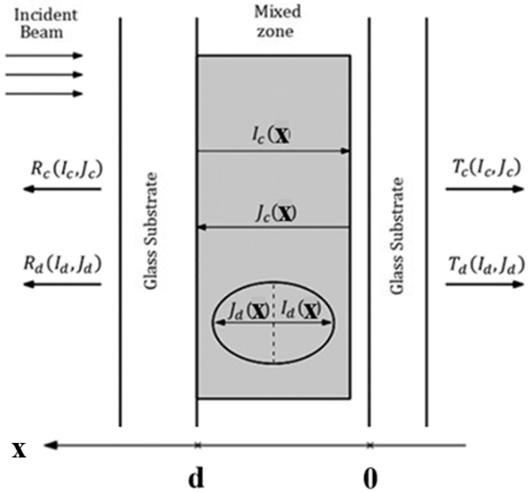Figure 4. Schema of the cell with different flux.

The four flux Ic, Id, Jc and Jd included in the previous equations, (7), (8), (9) and (10) are determined by taking into account the following boundary conditions.

At the Glass/Mixed zone: x=a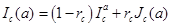(15)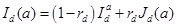(16)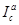and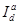I are the incident collimated flux and the incident diffuse flux at the interface x=a.can be determined by taking into account the interference effects at the glass/mixed zone and air/glass interfaces and is given as: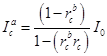(17)

In fact,is equal to zero and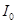is the incoming light.

At the Mixed zone/Glass: x=0

By considering the reflection effects at the air/glass and the glass/mixed interfaces the following conditions can be established: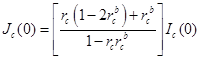(18)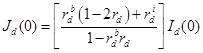(19)

where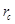,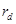,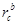,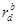the reflective coefficient of collimated beam, the reflective coefficient of radiation diffuse beam, the reflective coefficient of collimated beam at the back, the reflective coefficient of radiation diffuse beam at back side respectively.

Where: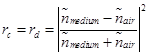(20)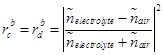(21)

Where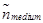is determined using Bruggeman theory.

Then, the 4-flux are: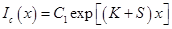(22)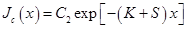(23)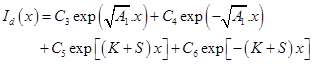(24)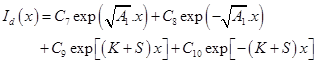(25)

Where A1, C1, C2, C3, C4, C5, C6, C7, C8, C9 and C10 function of K and S.

Thus, the local optical absorption rate per unit volume  can be established using the expression below: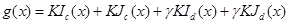(26)

Hence, the optical photons absorption rate is deduced from g(x) , and is expressed as: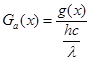(27)

And Gdye is deduced by: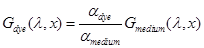(28)

Finally, the optical photo-generation rate of the DSC is given by: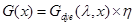(29)

where η being the injection rate of electrons.

2.2. Electrical Model

The charge generation function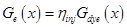, is coupled to an electrical model for free charge carriers. Here, for simplicity, we do not include ionic transport in the electrolyte and the reduction of triiodide at the counter-electrode. The electrical model is based on the stationary continuity equation. We suppose that there is no trapping states, i.e the electron is only in the conduction band of the TiO2 layer. A purely diffusive transport equation for the electrical current density J is taken . Then, in the ideal model case, only electrons from the conduction band can recombine with triiodide in the electrolyte, and the recombination rate is taken to be first order in n(x)(n(x): carriers density). This leads to a linear differential equation for n(x):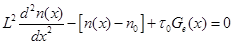(30)

Here,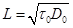is the constant electron diffusion length, τ is the electron lifetime, and n0 is the electron number density at equilibrium in the dark.

Since the porosity of the electrode influences the electron transport, the expression of the porosity of the electron diffusion coefficient D0 can be expressed as :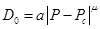for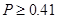(31)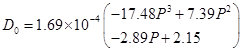(32)

for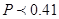Where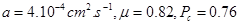The electron number density in the dark n0 is given by: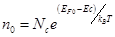(33)

where Nc is the effective density of the conduction band states, Ec is the conduction band energy, and EF0 is the Fermi level in the dark, which is equilibrated with the redox potential of the iodide/triiodide couple.

The boundary conditions to equation (31) are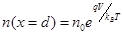(34)

And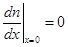(35)

where V is the photo-voltage.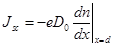for V=0 (36)

3. Results and Discussion

After calculating the analytical expressions presented in the previous section, a numerical code implemented with Matlab is established. The numerical results are presented in this section. Thus, the macroscopic parameters such as the generation rate, the photocurrent density and the maximum power output of a DSSC based on several parameters are studied: wavelengths of the incident light overlap distance between two TiO2 particles, the depth of the cell.

The analytical methods were validated by data found in the literature.

In figure 5, we plot the generation rate depending on the photoactive layer. We make a comparison between the values obtained by our model and the Beer-Lambert law. However, the optical method based on Mie theory and RTE provides a more detailed view as shown in the following paragraphs.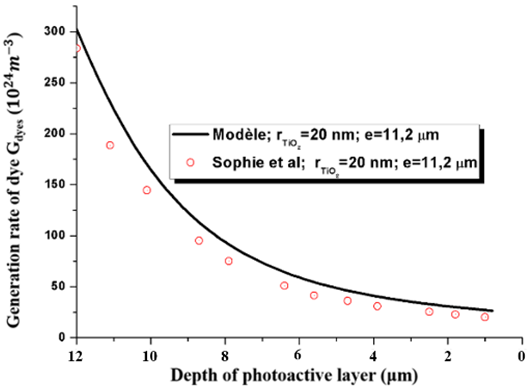Figure 5. Generation rate of photoactive layer as a function of the cell: Generation rate of photoactive layer as a function of the cell.

The modeling of the short-circuit photocurrent density is compared with the experimental results of Saito et al (figure 6) . The model and the experimental results show a good agreement.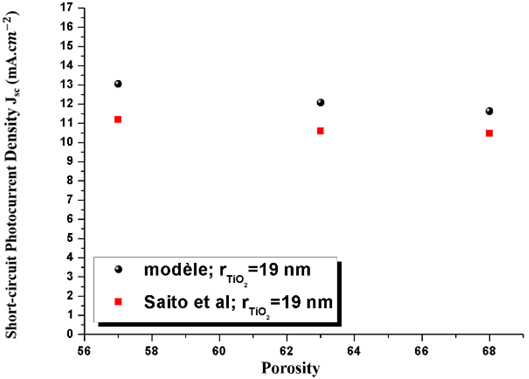Figure 6. Short circuit photocurrent density as a function of porosity.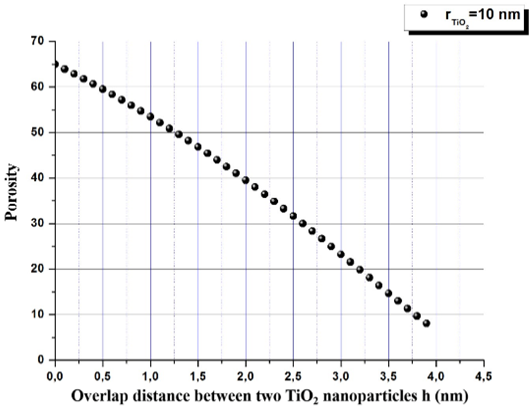Figure 7. Porosity depending on the overlap distance h.

Figure 7 shows the variation of porosity as a function of the overlap distance between two TiO2 particles h for a radius equal to 10 nm. We see that, when h increases, the porosity decreases. In fact, when h increases the package density increases, which lead to a reduction of the porosity.

Figure 8 represents the profile of the density of dyes as a function of TiO2 radius for different values of overlap distance between two TiO2 nanoparticles. We see that when the radius increases the density of dye decreases. In fact, when the radius increases the specific surface area is reduced. Moreover, we see that when the overlap distance is smaller than 2 nm (figure 8.a) the density of dyes is nearly the same. However, when the overlap distance is greater than 2 nm (figure 8.b) the density of dyes decreases. When the overlap distance is smaller than 2 nm, the specific surface area is proportional to the overlap distance. Thus, the increase of overlap distance causes a small reduction of the specific surface area. Then, the variation of the density of dyes is small. However, when the overlap distance is greater than 2 nm, the specific surface area is proportional to the square of the porosity. The reduction of the porosity causes a reduction of the specific surface area. Then, the reduction of surface area causes the decrease of density of dyes.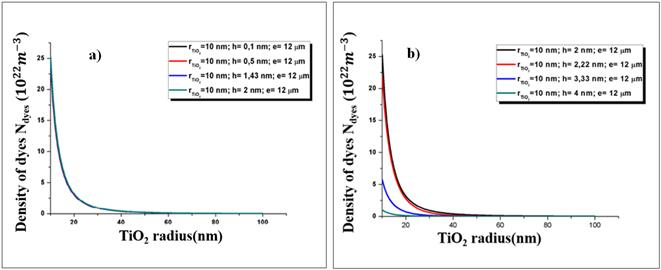Figure 8. Density of dye as a function of overlap distance between two TiO2 particles.

Figure 9 represents the generation rate as a function of the depth of the photoactive layer for different values of the overlap distance. We notice that the absorption of photons is greater at the interface anode/ photoactive layer. Indeed, the incident flux is reduced when the light passes through the photoactive layer. Moreover, as for figure 8, when the overlap distance is smaller than 2 nm (figure 8. a), we have a small reduction of the generation rate when the overlap distance increases. However, when the overlap distance is greater than 2 nm (figure 8. b), when the overlap distance increases the generation rate decreases. Indeed, as shown in figure 4, when the overlap distance is smaller than 2 nm, a growth of the overlap distance causes a small reduction of the dyes, thus a small reduction of generation rate. However, when the overlap distance is greater than 2 nm, an increase of the overlap distance causes a decrease of dyes, then a reduction of the generation rate.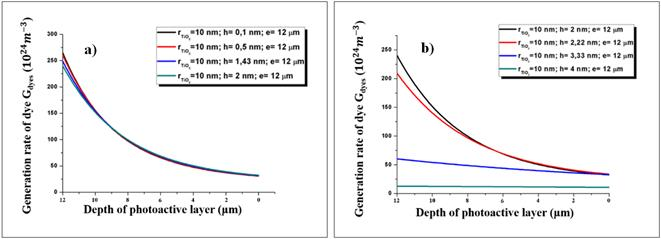Figure 9. Generation rate depending on the depth of the cell, for different values of h.

Figure 10 presents the diffusion coefficient of electrons as a function of overlap distance between two TiO2 nanoparticles h for a radius equal to 10 nm. When h increases, the diffusion coefficient increases. In fact, when h increases, the contact between particles increases, thus the diffusion between two particles increases. However, we notice that the curve changes when the overlap distance equals to 2 nm. In fact, the diffusion coefficient is described by two different equations as seen in the precedent section.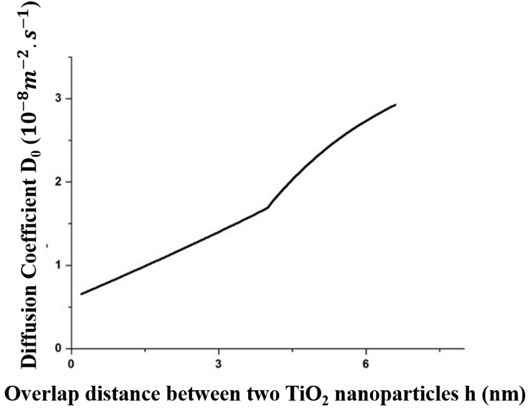Figure 10. Diffusion coefficient as a function of overlap distance.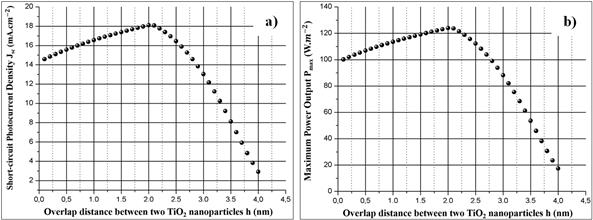Figure 11. Short-circuit photocurrent density and Maximum power output as a function of overlap distance.

Figure 11.a represents the short-circuit current density as a function of overlap distance. We notice that when the overlap distance h increases, the short circuit current density increases for h smaller than 2 nm, but the short circuit current density decreases when h greater than 2 nm with a maximum to h = 2 nm. Indeed, for h smaller than 2 nm, when the overlap distance increases the generation rate is almost the same (Cf figure 9.a) but the diffusion coefficient increases (Cf figure 10). Then, the short circuit current density increases. However, when h is smaller than 2 nm, when the overlap distance increases, the generation rate decreases (Cf figure 9.b). Then, even if the diffusion coefficient increases, we assist to a reduction of photon. Thus, short circuit current density decreases.

Figure 11.b represents the maximum power output as a function of overlap distance. We see that, we have the same behavior as for figure 11.a. Then, we notice that it exists an optimal overlap distance h = 2 nm, for TiO2 radius equal to 10 nm.

4. Conclusion

Our model allows us to see the influence of overlap distance between two TiO2 particles (h) on the generation rate. Moreover, the influence of h in the electric output parameters (Jsc, Pmax …) is studied.

We see that when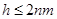the generation rate is nearly the same. However, when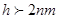the generation rate is reduced. Furthermore, it was found that the optimal overlap distance is 2 nm for for TiO2 radius equal to 10 nm.

Our results agree with the ones found in the literature. However, this model has some limitation: the particles are not necessarily spherical and of the same size and the difference between the reflected coefficient diffused and collimated could be taken into account for better precision.

References

1. O’Regan, B., Grätzel, M., ‘‘A low-cost, high-efficiency solar cell based on dye-sensitized colloidal TiO2 films,’’ Nature, 353-737, 1991.
2. Wang, Q., Ito, S., Gratzel, M., Fabregat-Santiago, F., Mora-Sero, I., Bisquert, J., Bessho, T., and Imai, H., ‘‘Characteristics of High Efficiency Dye-Sensitized Solar Cells,’’ J. Phys. Chem. B 110, 25210-25221, 2006.
3. Mathew, S., Yella, A., Gao, P., Humphry-Baker, R., Curchod, B. F. E., Tavernelli, I., Rothlisberger, U., Nazeeruddin M. K., and Grätzel, M., ‘‘Dye-sensitized solar cells with 13% efficiency achieved through the molecular engineering of porphyrin sensitizers,’’ Nature Chemistry 6, 242–247, 2014.
4. Green, M. A., Emery, K., Hishikawa, Y., Warta, W., and Dunlop, E. D., ‘‘Solar cell efficiency tables (Version 45),’’ Progress in photovoltaics: research and applications, 23 (1), 1-9, 2015.
5. Chiba, Y.; Islam, A.; Watanabe, Y.; Komiya, R.; Koide, N.; Han, L., J. Appl. Phys., Part 2, 45, L638–L640, 2006.
6. Gao, F.; Wang, Y., Shi, D., Zhang, J., Wang, M. K., Jing, X. Y., Humphry-Baker, R., Wang, P., Zakeeruddin, S. M., Grätzel, M., J. Am. Chem. Soc. 130, 10720–10728, 2008.
7. Ferber, J., and Luther, J., ‘‘Computer simulations of light scattering and absorption in dye-sensitized solar cells.’’ Solar Energy Materials and Solar Cells 54 (1998)
8. Rothenberger, G., Comte, P., Gratzel, M., ‘‘A contribution to the optical design of dye_sensitized nanocrystalline solar cells,’’ Solar Energy Materials & Solar Cells, 58, 321-336, 1999.
9. Soedergren, S., Hagfeldt, A., Olsson, J., and Lindquist, S. E., ‘‘Theoretical models for the action spectrum and the current-voltage characteristics of microporous semiconductor films in photoelectrochemical cells,’’ The Journal of Physical Chemistry, 98 (21), 5552-5556, 1994.
10. Matthews, D., Infelta, P., and Grätzel, M, ‘‘Calculation of the photocurrent-potential characteristic for regenerative, sensitized semiconductor electrodes.’’ Solar Energy Materials and Solar Cells, 44 (2), 119-155, 1996.
11. Ferber, J., Stangl, R., and Luther, J., "An electrical model of the dye-sensitized solar cell," Solar Energy Materials and Solar Cells, 53 (1), 29-54, 1998.
12. Usami, A., "Theoretical study of application of multiple scattering of light to a dye-sensitized nanocrystalline photoelectrichemical cell," Chemical Physics Letters, 277 (1), 105-108, 1997.
13. Usami, A., "Theoretical study of charge transportation in dye-sensitized nanocrystalline TiO2 electrodes," Chemical physics letters, 292 (1), 223-228, 1998.
14. Ferber, J., Stangl, R., and Luther, J.,"An electrical model of the dye-sensitized solar cell,"Solar Energy Materials and Solar Cells, 53 (1), 29-54, 1998.
15. Stangl, R., Ferber, J., & Luther, J., ‘‘On the modeling of the dye-sensitized solar cell,’’ Solar Energy Materials and Solar Cells, 54 (1), 255-264, 1998.
16. Usami, A., & Ozaki, H., ‘‘Computer simulations of charge transport in dye-sensitized nanocrystalline photovoltaic cells,’’ The Journal of Physical Chemistry B, 105 (20), 4577-4583, 2001.
17. Bisquert, J., Cahen, D., Hodes, G., Rühle, S., & Zaban, A., ‘‘Physical chemical principles of photovoltaic conversion with nanoparticulate, mesoporous dye-sensitized solar cells,’’ The Journal of Physical Chemistry B, 108 (24), 8106-8118, 2004.
18. Filipič, M., Berginc, M., Smole, F., & Topič, M., ‘‘Analysis of electron recombination in dye-sensitized solar cell,’’ Current Applied Physics, 12 (1), 238-246, 2012.
19. Wenger, S., Schmid, M., Rothenberger, G., Gentsch, A., Gratzel, M., and Schumacher, J. O., ‘‘Coupled Optical and Electronic Modeling of Dye-Sensitized Solar Cells for Steady-State Parameter Extraction,’’ J. Phys. Chem. C 115, 10218–10229, 2011.
20. Topič, M., Čampa, A., Filipič, M., Berginc, M., Krašovec, U. O., & Smole, F., ‘‘Optical and electrical modelling and characterization of dye-sensitized solar cells,’’ Current Applied Physics, 10 (3), S425-S430, 2010.
21. Taylor, S. W. ‘‘Transport of substrate and biomass in porous media with application to in situ bioremediation of organic contaminants in groundwater,’’ PhD thesis, Department of Civil Engineering, Princeton, 1990.
22. Taylor, N. J., Milly, S. W., and Jaffe, P. R., ‘‘Biofilm growth and the related changes in the physical properties of a porous medium, 2, Permeability,’’ Water Resour. Res., 26 (9), 2161–2169, 1990.
23. Deb, A. K. ‘‘Theory of sand filtration.’’J. Sanit. Eng. Div., ASCE, 96 (3), 399–422. (1969).
24. B. Maheu, J. N. Letoulouzan and G. Gouesbet. Four-flux models to solve the scattering transfer equation in terms of Lorenz-Mie parameters. Applied Optics Vol. 23, No. 19 (1984).
25. G. Kortum, Reflectance Spectroscopy, Springer, Berlin, 1969.
26. C. Rozé, T. Girasole, G. Gréhan, G.Gouesbet, B. Maheu. Four-flux models to solve the scattering transfer equation in terms of Lorenz-Mie parameters. Optics communications 194 (2001) 251-263.
27. A. Dioum, S. Ndiaye, E. H. O. Gueye, M. B. Gaye, D. N. Faye, O. Sakho, M. Faye and A. C. Beye. 3-D Modeling of bilayer heterojunction organic solar cell based on Copper Phthalocyanine and Fullerene (CuPc/C60): evidence of total excitons dissociation at the donor-acceptor interface. Global Journal of Pure and Applied Sciences, Vol 19 (2013).
28. Benkstein, K. D., Kopidakis, N., van de Lagemaat, J., Frank, A. J., ‘‘Influence of the Percolation Network Geometry on Electron Transport in Dye-Sensitized Titanium Dioxide Solar Cells,’’ Phys. Chem. B, 107-114, 2003.
29. Saito, Y., Kambe, S., Kitamura, T., Wada, Y., and Yanagida, S., ‘‘Morphology control of mesoporous TiO2 nanocrystalline films for performance of dye-sensitized solar cells,’’ Solar Energy Materials and Solar Cells, 83 (1), 1-13, 2004.

 Contents 1. 2. 2.1. 2.2. 3. 4.
Article ToolsAbstractPDF(734K)